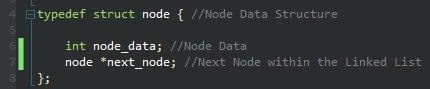## Monday, 20 January 2014

### Algorithms and Data Structures - Calculating Insertion and Deletion Time Complexity of Singly Linked Lists

Prerequisites:
- Knowledge of C/C++
- Knowledge of Calculus/Algebra

Time Complexity and O(n)

You could consider this topic as a Computer Science/Programming topic. However, I always consider Computer Science and Programming to be two different topics rather than the same thing, even though they both share the same programming principles, such as understanding how to write code to begin with. Computer Science is more like Applied Mathematics, and is more theory based, whereas, Programming is more practical and using a language or multiple languages as tools to create real-life programs.

I've written a simple program which creates nodes within a linked list, and then walks this linked list when the user has decided they do not wish to insert any more nodes into the list. I've commented the code where necessary so it will be easier to understand. We'll then calculate the time complexity of the algorithm used to insert the nodes within the linked list.

Firstly, let's consider and investigate the concept of time complexity and what it means in terms of Computer Science. Time Complexity refers to the amount of time for a operation to complete, as a result of the input required. There are different forms of Time Complexity, and therefore different methods apply to the type of Time Complexity. For Singly Linked Lists, the Time Complexity is often regarded as Constant Time Complexity.

Constant Time Complexity or simply Constant Time, isn't affected by the size of the input, or the number of elements. For example, if we had a sorted linked list or array, it would only take one operation to find the element within the array. Although, the same can still apply, if the number of elements within the linked list was unsorted, but the number of elements within the linked list is known within advance. To simply, the number of elements within the linked list, does not affect the complexity of the insertions or deletions within the linked list. The Big O Notation for calculating constant time within a linked list is O(1). On the other hand, the Big O Notation for  walking the list, is given by the O(n), which implies that the complexity for walking the list, increases with direct proportion to the number of elements within the linked list.

The Big-O Complexity Interactive Graph supports this point.Figure 1 - Node Structure
Let's examine this simple node structure. The structure has two members: node_data and next_node. The node_data is of a integer type, and represents any data stored within each node. The next_node is a pointer with a type of node (structure), this is used to point to the next node within the singly linked list.Figure 2 - create_node() Function

The function will keep creating new nodes as long as the user wants to. The node_data can be defined by the user.Figure 3 - create_node() Function
Once, the user has created all the desired nodes within the linked list, the walk_list function will be called.

References

Big O Notation
Time Complexity
Complexity Theory - Definitions of an algorithm running in polynomial time and strongly polynomial time
A Beginner's Guide to Big O Notation
How To Create a Linked List Using C/C++

1.2.